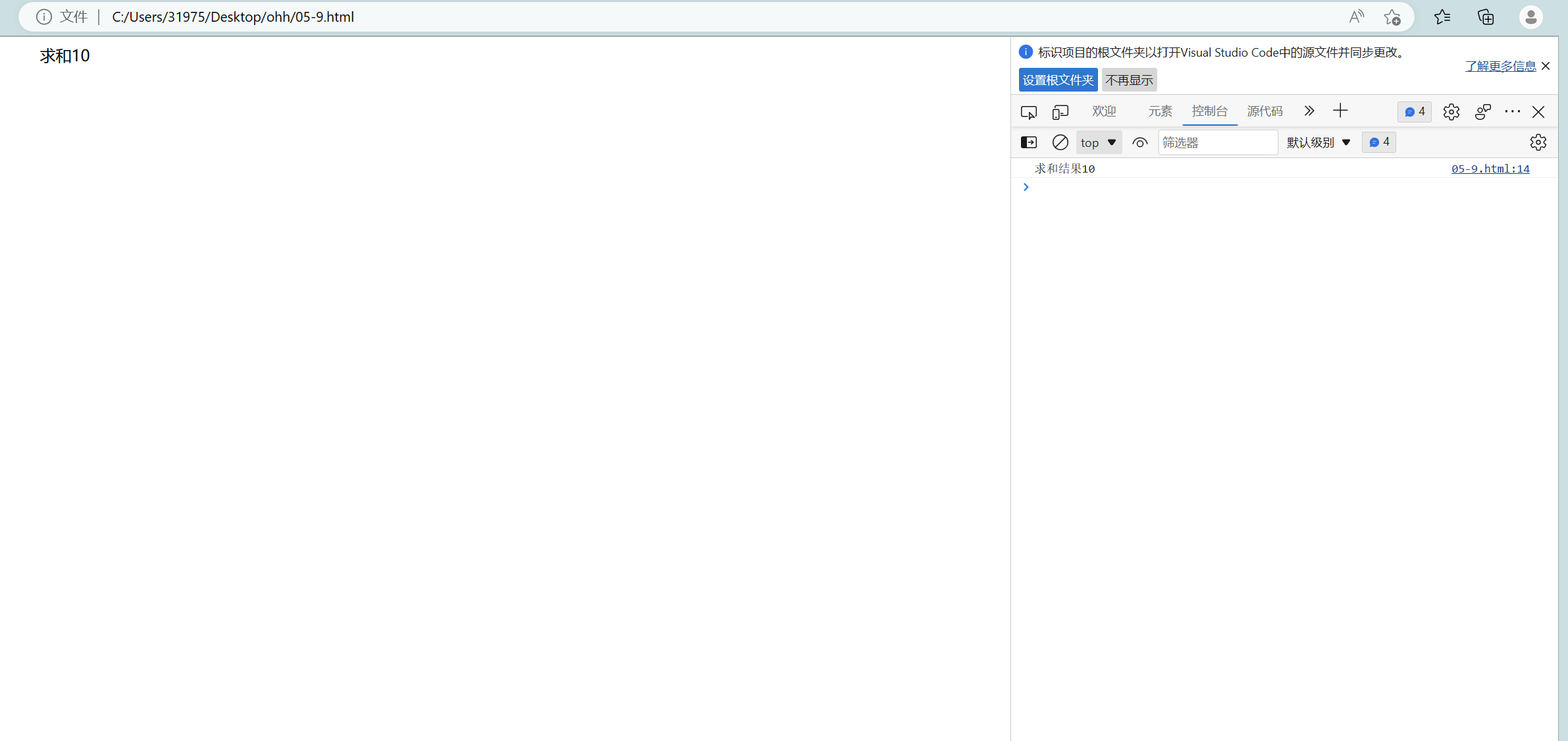# 第三次作业<head>
<body>
<script>
//输入方式
var num1;
num1 = parseInt(prompt("请输入第一个要进行运算的数据"));
var num2;
num2 = parseInt(prompt("请输入第二个要进行运算的数据"));
var sum = num1 + num2;
//输出方式
document.write("求和"+sum);
//在控制台输出信息
console.log("求和结果"+sum);
</script>
</body>
</html>